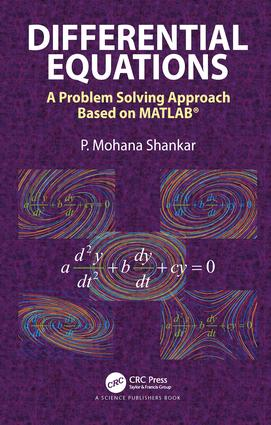Differential Equations

A Problem Solving Approach Based on MATLAB, 1st Edition

CRC Press

448 pages | 227 B/W Illus.

Purchasing Options:\$ = USD
Hardback: 9781138501607
pub: 2018-03-28
SAVE ~\$37.00
\$185.00
\$148.00
x
eBook (VitalSource) : 9781315144429
pub: 2018-04-17
from \$28.98

FREE Standard Shipping!

Description

The book takes a problem solving approach in presenting the topic of differential equations. It provides a complete narrative of differential equations showing the theoretical aspects of the problem (the how's and why's), various steps in arriving at solutions, multiple ways of obtaining solutions and comparison of solutions. A large number of comprehensive examples are provided to show depth and breadth and these are presented in a manner very similar to the instructor's class room work. The examples contain solutions from Laplace transform based approaches alongside the solutions based on eigenvalues and eigenvectors and characteristic equations. The verification of the results in examples is additionally provided using Runge-Kutta offering a holistic means to interpret and understand the solutions. Wherever necessary, phase plots are provided to support the analytical results. All the examples are worked out using MATLAB® taking advantage of the Symbolic Toolbox and LaTex for displaying equations. With the subject matter being presented through these descriptive examples, students will find it easy to grasp the concepts. A large number of exercises have been provided in each chapter to allow instructors and students to explore various aspects of differential equations.

Reviews

"It introduces the basic theoretical notions and methods concerning the aforementioned topics and provides the MATLAB tools and codes for solving the basic classes of ordinary differential equations(ODEs) and systems of ODEs: rst order differential equations (DEs), second order linear DEs with constant coe?cients, higher-order linear DEs with constant coeffi?cients, systems of first order DEs with constant coe?cients. There are considered especially the issues of graphical representation for time evolution, state space, qualitative analysis of the equilibrium points, vector eld representation."

-- Daniela Danciu (Craiova). Zentralblatt MATH 1406

First Order Differential Equations

Introduction

D-field plots

Methods of solving first order differential equations

Additional examples on D-field plots

Autonomous Differential Equations

Summary

Exercises

Linear Second Order Differential Equations with constant Coefficients

Introduction

Homogeneous Differential Equations

Non-homogeneous Differential Equations: Particular Solutions and Complete Solutions

Summary

Exercises

Linear Higher Order Differential Equations with Constant Coefficients

Introduction

Homogeneous differential equations (n<5)

Non-homogeneous Differential equations and Particular solutions (n<5)

Additional methods of obtaining the solution and verification

Higher order differential equations (n>4)

Examples

Summary

Exercises

First order coupled differential equations with constant coefficients

Introduction

A pair of coupled differential equations

Multiple first order coupled differential equations of constant coefficients

Numerical Solutions

Examples

Summary

Exercises

Professor P. Mohana Shankar received his B.Sc. (Physics) and M.Sc. (Physics) from Kerala University and M. Tech. (Applied Optics) and Ph. D. (Electrical Engineering) from the Indian Institute of Technology, Delhi, India. He was the William Girling Watson visiting scholar at the School of Electrical Engineering, University of Sydney, Australia from 1981-1982. He has been at Drexel University since November 1982. He started as a postdoctoral fellow in Biomedical Engineering and since 2001, he is the Allen Rothwarf Professor in the department of Electrical and Computer Engineering. He has more than 100 archival journal publications covering the fields of nonlinear acoustics, ultrasonic contrast agents, ultrasonic non-destructive testing, ultrasonic tissue characterization, statistical signal processing, medical statistics, fiberoptic communications, fiberoptic biosensors, wireless communications, modeling of fading and shadowing wireless channels, etc. His publications also include work in pedagogy of differential equations, linear algebra, wireless communications, and engineering probability, all with emphasis on the use of Matlab (www.mathworks.com). He is the author of three books in wireless communications.